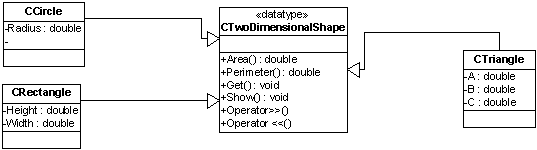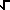# OOP  - Assignment 7

Topic:              Inheritance-Polymorphism-Virtual-Functions

Points:             25

### Due: 10-28-2003

Instructor:      Dana Steil• The Class TwoDimensionalShape should be an abstract base class.
• All member functions of TwoDimensionalShape should be pure virtual.
• The get and the show functions should be private the rest should be public.
• The friend stream insertion and the stream extraction operators should call the show and get.
• The other classes should inherent from TwoDimensionalShape.
• Do not worry with any data validation.

Use the following test program.  This test program will be used to test your assignment.

enum eShape

{

CIRCLE = 0,

RECTANGLE,

TRIANGLE

};

istream& operator>> (istream& input, eShape& Shape)

{

char UserResponse;

do

{

cout << "Do you want to create a (C)ircle (R)ectangle or (T)riangle? ";

cin >> UserResponse;

UserResponse = toupper(UserResponse);

}

while(UserResponse != 'C' && UserResponse != 'R' && UserResponse != 'T');

switch (UserResponse)

{

case 'C':

Shape = CIRCLE;

break;

case 'R':

Shape = RECTANGLE;

break;

case 'T':

Shape = TRIANGLE;

break;

}

return input;

}

void GetShapes(CTwoDimensionalShape* Shapes[])

{

eShape Shape = CIRCLE;

for (int i = 0; i < TOTAL_SHAPES; i++)

{

cin >> Shape;

switch (Shape)

{

case CIRCLE:

Shapes[i] = new CCircle;

break;

case RECTANGLE:

Shapes[i] = new CRectangle;

break;

case TRIANGLE:

Shapes[i] = new CTriangle;

break;

}

cin >> *Shapes[i];

}

}

void ShowShapes(CTwoDimensionalShape* const Shapes[])

{

for (int i = 0; i < TOTAL_SHAPES; i++)

{

cout << *Shapes[i];

}

}

void DeleteShapes(CTwoDimensionalShape* Shapes[])

{

for (int i = 0; i < TOTAL_SHAPES; i++)

{

if (Shapes[i] != NULL)

{

delete Shapes[i];

Shapes[i] = NULL;

}

}

}

void main()

{

CTwoDimensionalShape* Shapes[TOTAL_SHAPES];

GetShapes(Shapes);

ShowShapes(Shapes);

DeleteShapes(Shapes);

}

 Area Perimeter Circle PI * Radius2 2 * Radius * PI Rectangle Base * Height 2 * Base + 2 * Height Triangle[s(s-a)(s-b)(s-c)] when s = (a+b+c)/2 (Heron's formula) A + B + C